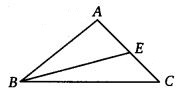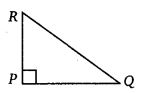# Class 7 Maths NCERT Solutions for Chapter – 6 The Triangle and its Properties Ex – 6.1

### The Triangle and its Properties

Question 1.

Solution:

Question 2.
Draw rough sketches for the following :
(a)
In ∆ABC, BE is a median.
(b) in ∆PQR, PQ and PR are altitudes of the triangle.
(c) In ∆XYZ, YL is an altitude In the exterior of the triangle.

Solution:
(a) Rough sketch of median BE of ∆ABC is as shown.(b) Rough sketch of altitudes PQ and PR of ∆PQR is as shown.(c) Rough sketch of an exterior altitude YL of ∆XYZ is as shown.Question 3.
Verify by drawing a diagram if ‘the median and altitude of an isosceles triangle can be same.

Solution:Draw a line segment BC. By paper folding, locate the perpendicular bisector of BC. The folded crease meets BC at D, its mid-point.

Take any point A on this perpendicular bisector. Join AB and AC. The triangle thus obtained is an isosceles ∆ABC in which AB = AC.

Since, D is the mid-point of BC, so AD is its median. Also AD is the perpendicular bisector of BC. So, AD is the altitude of ∆ABC.

Thus, it is verified that the median and altitude of an isosceles triangle are same.